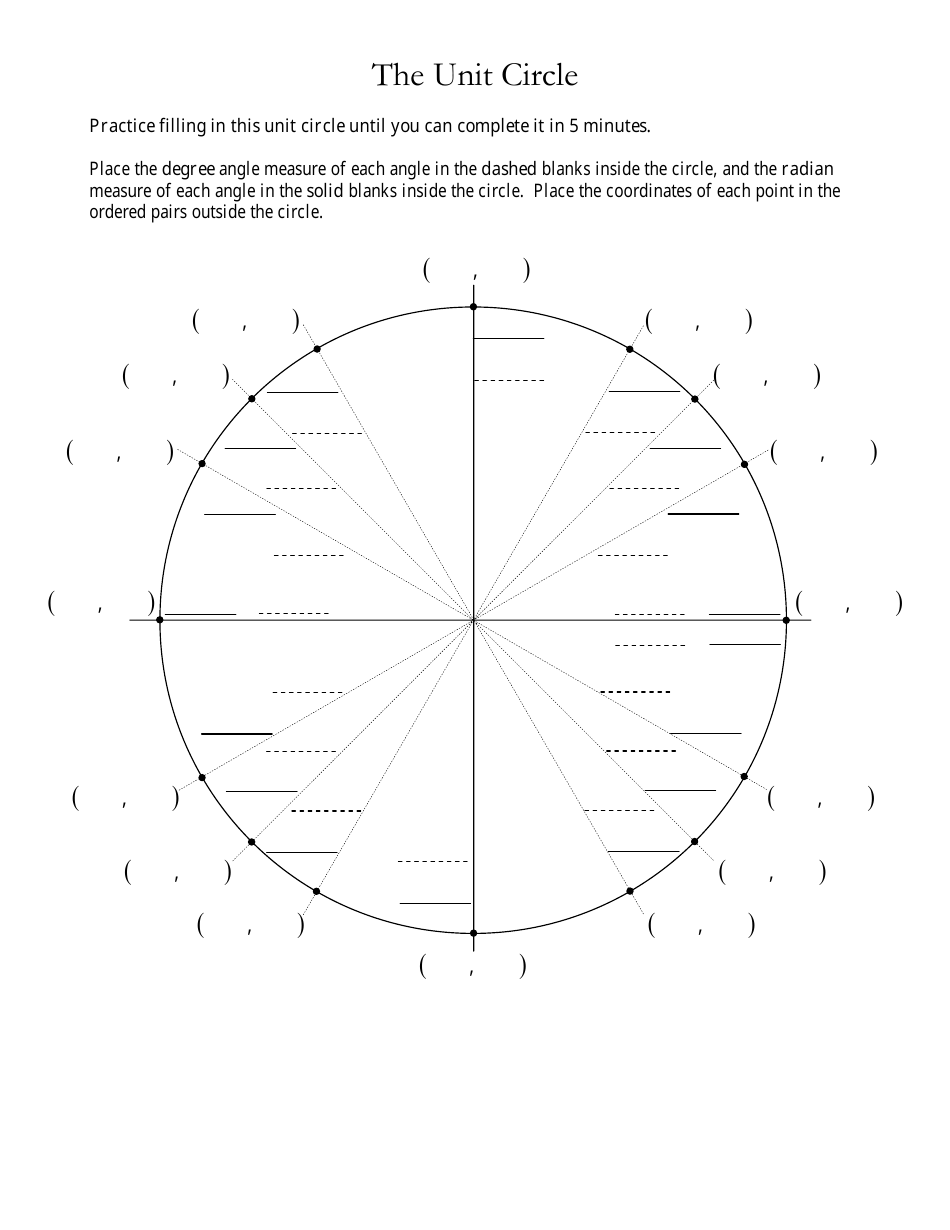# Unit Circle Worksheet

A unit circle worksheet is a practice tool used to understand and solve math problems related to the unit circle. It helps students visualize and understand the properties of angles and trigonometric functions on the unit circle.

The unit circle worksheet is typically filed by the teacher or instructor who assigns it to the students.

## FAQ

Q: What is a unit circle?
A: A unit circle is a circle with a radius of 1 unit.

Q: What is the purpose of a unit circle?
A: The unit circle is used in trigonometry to relate the angles to the coordinates on the circle.

Q: How do you find the coordinates of a point on the unit circle?
A: The coordinates of a point on the unit circle are (cosine(angle), sine(angle)).

Q: What are the special angles on the unit circle?
A: The special angles on the unit circle are 0°, 30°, 45°, 60°, and 90°.

Q: What are the values of sine and cosine for the special angles on the unit circle?
A: For 0°, cosine = 1 and sine = 0; for 30°, cosine = √3/2 and sine = 1/2; for 45°, cosine = √2/2 and sine = √2/2; for 60°, cosine = 1/2 and sine = √3/2; for 90°, cosine = 0 and sine = 1.

•